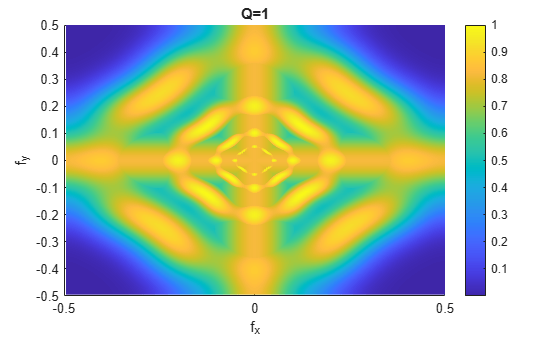# littlewoodPaleySum

Littlewood-Paley sum

## Syntax

``lpsum = littlewoodPaleySum(sf)``
``lpsum = littlewoodPaleySum(sf,fb)``
``[lpsum,f] = littlewoodPaleySum(___)``

## Description

````lpsum = littlewoodPaleySum(sf)` returns the Littlewood-Paley sum for the 2-D filter banks in the 2-D wavelet scattering network `sf`. `lpsum` is an M-by-N-by-Nfb matrix, where M-by-N is the matrix size of the padded filters and Nfb is the number of filter banks.Since the scattering transform is contractive, the Littlewood-Paley sums do not exceed 1.```
````lpsum = littlewoodPaleySum(sf,fb)` returns the Littlewood-Paley sum for the specified filter banks `fb`. `fb` is a positive integer or vector of positive integers between 1 and `numfilterbanks(sf)` inclusive. `lpsum` is an M-by-N-by-L matrix, where L is the number of unique elements in `fb`.```

example

````[lpsum,f] = littlewoodPaleySum(___)` returns the spatial frequencies for the Littlewood-Paley sum. `f` is a two-column matrix with the first column containing the spatial frequencies in the x-direction and the second column containing the spatial frequencies in the y-direction.```

## Examples

collapse all

This example shows how to obtain and display the Littlewood-Paley sum of an image scattering network.

Create a scattering network with two filter banks and quality factors of 2 and 1, respectively.

`sf = waveletScattering2('QualityFactors',[2 1]);`

Obtain the Littlewood-Paley sums and spatial frequencies of the two filter banks. Display the maximum value of the sums. Since the scattering transform is contractive, the sums do not exceed 1.

```[lpsum,f] = littlewoodPaleySum(sf); max(max(lpsum(:,:,1)))```
```ans = 1.0000 ```
`max(max(lpsum(:,:,2)))`
```ans = 1.0000 ```

Display the Littlewood-Paley sum of the second filter bank with the zero frequency centered. Note the 2-D Morlet filter bank used in the scattering transform is not designed to capture the highest spatial frequencies jointly in the x- and y-directions.

```f(f>1/2) = f(f>1/2)-1; surf(fftshift(f(:,1)),fftshift(f(:,2)),fftshift(lpsum(:,:,2))) shading interp view(0,90) xlabel('f_x') ylabel('f_y') colorbar title('Q=1')```## Input Arguments

collapse all

Wavelet image scattering network, specified as a `waveletScattering2` object.

Filter bank index in the image scattering network, specified as a positive integer or vector of positive integers between 1 and `numfilterbanks(sf)` inclusive. The number of filter banks in `sf` is equal to the number of specified `QualityFactors` in `sf`.

## Output Arguments

collapse all

Littlewood-Paley sum for the filter banks in the image scattering network `sf`, returned as a real-valued 3-D matrix. `lpsum` is an M-by-N-by-L matrix, where M-by-N is the matrix size of the padded filters and L does not exceed the number of filter banks in `sf`.

Frequencies for the Littlewood-Paley sum, returned as a real-valued two-column matrix. Frequencies are in cycles per pixel. The first column of `f` contains the spatial frequencies in the x-direction, and the second column contains the spatial frequencies in the y-direction. In this convention, the Fourier transform is 1-periodic in both Fourier variables.